# Electroscope with Examples

Electroscope

It is a device that is used for detecting whether an object is charged or uncharged. It is also determine the type of charge.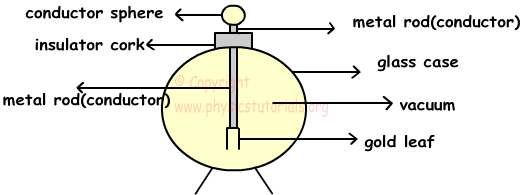As you can see from the picture there is a metal (conductor sphere) and a metal rod attached to this sphere. Gold leaves are at the bottom of the rod. Electroscopes are placed in a glass case to diminish the effects of wind and ions in the air. This picture shows the neutral electroscope. If we charge it, because of the fact that same charges repel each other, leaves of the electroscope rises. Amount of the distance between the leaves depends on the amount of charges electroscope has. We analyze the different type of charging electroscope below.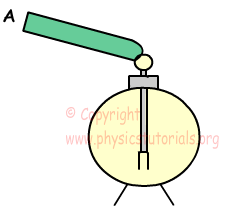In this example, conductor A touches to the sphere of the neutral electroscope, however leaves of it stay closed. We understand that A is neutral; if it was not neutral leaves would rise.

Here, as you can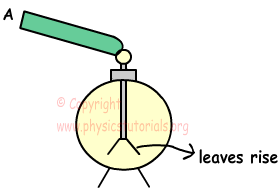see leaves of the electroscope rise with the coming charges from the conductor A. We do not know the type of charge but we can say that A is charged, not neutral. Below examples show how we can determine the types of charges.

To understand the type of charge of conductor, we should bring it closer to the charged electroscope.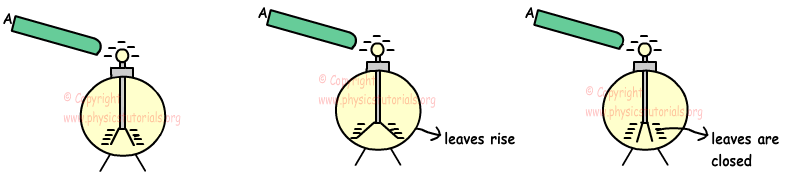Pictures given above shows the behavior of the leaves of negatively charged electroscope under the effect of charged rod. In the first picture, we bring rod closer to the sphere of negatively charged electroscope to observe motion of leaves. If the rod A is negatively charged , then same charges repel each other, electrons at the sphere are repelled to the end of the electroscope (to the leaves), and leaves rise with excess amount of electrons. Second picture shows this assumption. In the third picture, we assume that if the rod is positively charged, then rod attracts some of the electrons to the sphere and amount of electron on the leaves decreases. This decrease in the number of electrons makes them close. To sum up; when you bring charged object closer to the charged electroscope.

· If the leaves rise then both the rod and electroscope have same charge.

· But if the leaves are closed than charges of electroscope and rod are opposite.

Now we will examine the behavior of leaves when we touch charged object to the charged electroscope. There are different situations in this case, let’s look them one by one (we assume that capacities of electroscope and rod are same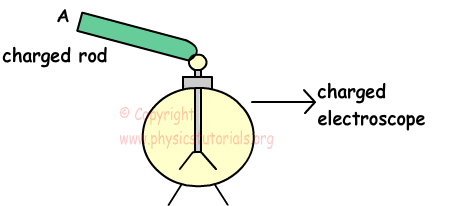1. If A has +q charge and electroscope has –q charge, total charge becomes zero and electroscope and rode becomes neutral and leaves are closed.

2. If A has +3q charge and electroscope has -4q, total charge becomes –q. Electroscope and rod share –q charge, thus, leaves of electroscope are closed a little bit. Be careful, they are not closed totally.

3. If A has +4q charge and electroscope has -2q charge, total charge of the system becomes +2q. Electroscope and rod share +2q charge, electroscope becomes positively charged. During this process, leaves of electroscope are closed and after completion of charge sharing they rise again.

Example: Positively charged electroscope and Y-Z plates are placed in the figure given below. When we put T conductor sphere next to the Y-Z plates, distance between the leaves of electroscope increases. Find the type of charges of the Y, Z and T.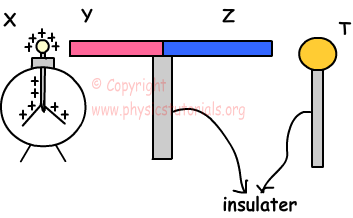Y-Z plates are charged by induction when T conductor placed next to them. To increase the distance between the leaves of the electroscope, it must be under the effect of same charge. So, Y must be +, to make leaves rise more. Moreover, if Y is +, then Z must be -, because opposite charges repel each other (electroscope attracts the electrons of the Y-Z plates to the left). Finally, if Z is -, then T is automatically becomes + to attract them.

Example: Negatively charged electroscope and X-Y plates are placed like in the figure given below. If the distances between electroscope and conductor plates are equals; find the types of charges of plates for given situations.

a) If there is no change in the distance between the leaves

b) If the leaves are closed a little bit

c)   If the leaves rise a little bit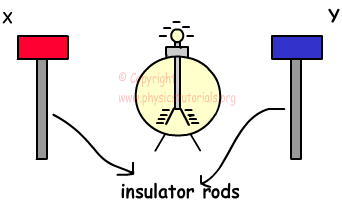a) If there is no change in the behavior of the leaves, then effect of one plate is canceled by other plate. This can be possible under the circumstances, amount of charge of X is equal to amount of charge of Y and one of them must be negatively charged and other one must be positively charged.

b) If the leaves are closed a little bit, then some of the electrons on the leaves must be move to the top of the electroscope. This can be possible with following ways;

1. Both of the plates can be positively charged and have different amounts of charges. They both attract electrons of the leaves.

2. They can have different charges; however, amount of positive charge must be larger than the negative charge. Since, there must be net positive charge to attract electrons from the leaves.

c) If the leaves rise a little bit, then some of the electrons from the top move to the leaves. This can be possible with following ways;

1. Both of the plates can be negatively charged and have different amounts of charges. They repel electrons from top of the electroscope to the leaves.

2. They can have different types of charges, but amount of negative charge must be larger than the amount of positive charge to repel electrons from top of the electroscope to the leaves.

Special Cases

Look at the given picture; when we put a charged conductor sphere into the neutral hollow, it is charged by induction. Negative charges are repelled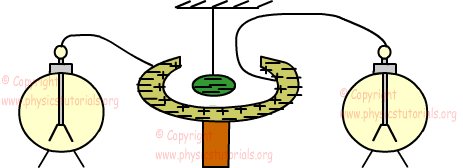to the outer part and positive charges stay at inner part. We connect neutral electroscopes to the inner and outer part of the hollow. Lea ves of neutral electroscopes rise that shows us it is charged by induction.When we touch negatively charged sphere into the inner part of hollow, we observe that sphere becomes neutral and electrons are repelled to the outer part of hollow. If we connect neutral electroscope to the outer part of the hollow, leaves of it rises, since outer part of hollow is negatively charged by contact. Be careful, inner part of hollow and sphere are becomes neutral.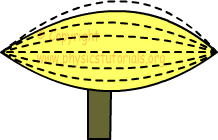Picture given below shows the charged conductor. As you can see, same charges repel each other and they are located as far as possible to each other. Density of charges at left and right points of the conductor is larger than the middle part.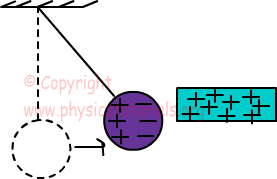Charged plate attracts the neutral sphere. Here we see the example of charging by induction. Electrons move to the right side of the sphere and attraction force is larger than the repulsion, thus sphere moves toward to the plate. If both plate and sphere are charged with same types of charge they repel each other. In the next section we will learn electrostatics forces between the charges and find the amount of forces.

Electrostatics Exams and Solutions

Related